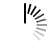# Print Matrix in spiral way

This algorithm is used to print the array elements in a spiral way. At first starting from the first row, print the whole content and then follow the last column to print, then the last row and so on, thus it prints the elements in spiral fashion.

The time complexity of this algorithm is O(MN), M is the number of rows and N is the number of columns.

#### Input and Output

```Input:
The matrix:
1   2   3   4   5   6
7   8   9  10  11  12
13  14  15  16  17  18

Output:
Contents of an array as the spiral form
1 2 3 4 5 6 12 18 17 16 15 14 13 7 8 9 10 11 15 16```

#### Algorithm

`dispSpiral(mat, m, n)`

Input: The matrix mat, row and column m and n.

Output: Print the elements of the matrix in a spiral way.

```Begin
currRow := 0 and currCol := 0
while currRow and currCol are in the matrix range, do
for i in range currCol and n-1, do
display mat[currRow, i]
done

increase currRow by 1
for i in range currRow and m-1, do
display mat[i, n-1]
done

decrease n by 1
if currRow < m, then
for i := n-1 down to currCol, do
display mat[m-1, i]
done
decrease m by 1
if currCol < n, then
for i := m-1 down to currRow, do
display mat[i, currCol]
done
increase currCol by 1
done
End```

#### Source Code (C++)

```#include <iostream>
#define ROW 3
#define COL 6
using namespace std;

int array[ROW][COL] = {
{1, 2, 3, 4, 5, 6},
{7, 8, 9, 10, 11, 12},
{13, 14, 15, 16, 17, 18}
};

void dispSpiral(int m, int n) {
int i, currRow = 0, currCol = 0;
while (currRow < ROW && currCol <COL) {
for (i = currCol; i < n; i++) {          //print the first row normally
cout << array[currRow][i]<<" ";
}
currRow++;           //point to next row

for (i = currRow; i < m; ++i) {       //Print the last column
cout << array[i][n-1]<<" ";
}

n--;               //set the n-1th column is current last column

if ( currRow< m) {         //when currRow is in the range, print the last row
for (i = n-1; i >= currCol; --i) {
cout << array[m-1][i]<<" ";
}
m--; //decrease the row range
}

if (currCol <n) {      //when currCol is in the range, print the fist column
for (i = m-1; i >= currRow; --i) {
cout << array[i][currCol]<<" ";
}
currCol++;
}
}
}

int main() {
dispSpiral(ROW, COL);
}```

#### Output

`1 2 3 4 5 6 12 18 17 16 15 14 13 7 8 9 10 11 15 16`